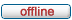# forum.alglib.net

ALGLIB forum
 It is currently Mon Sep 26, 2022 10:32 am

 All times are UTC

### Forum rules

1. This forum can be used for discussion of both ALGLIB-related and general numerical analysis questions
2. This forum is English-only - postings in other languages will be removed.

 Print view Previous topic | Next topic
Author Message
 Post subject: Needing help with Levenberg-MarquardtPosted: Tue Aug 05, 2014 6:10 amJoined: Mon Aug 04, 2014 12:17 pm
Posts: 2
Hi,
I'm quite new to this and so I'm currently struggeling a little bit with using "minlmcreatevj".

I want to fit a circle to a number of points. P(x,y)

I want to minimize the function: SUM[ ((xi-a)^2 + (yi-b)^2 - r^2)^2 ]
The documentation says, that i have to split it in single function f^2+f^2+f^2

I'm not sure if I did this right so here are my functions:

Code:
public static void function1_fvec(double[] x, double[] fi, object obj)
{
// m_x = x-coordinate of center
// m_y = y-coordinate of center
// r      = radius of circle
// x      = x-coordinate of point
// y      = y-coordinate of point

fi = Math.Pow(x - m_x, 2);
fi = Math.Pow(y - m_y, 2);
fi = -Math.Pow(r, 2);
}

public static void function1_jac(double[] x, double[] fi, double[,] jac, object obj)
{
// m_x = x-coordinate of center
// m_y = y-coordinate of center
// r      = radius of circle
// x      = x-coordinate of point
// y      = y-coordinate of point

fi = Math.Pow(x - m_x, 2);
fi = Math.Pow(y - m_y, 2);
fi = -Math.Pow(r, 2);

jac[0, 0] = -2 * (x - m_x);
jac[0, 1] = 0;
jac[0, 2] = 0;
jac[1, 0] = 0;
jac[1, 1] = -2 * (y - m_y);
jac[1, 2] = 0;
jac[2, 0] = 0;
jac[2, 1] = 0;
jac[2, 2] = -2 * r
}

But I don't understand, where I can input my points. Otherwise he wouldn't know to what points he should fit the circle.
I'm using C#.

I'm a little bit confused and I hope, that you could help me with my problem how this library actually works.

Best regards

Dominik

TopPost subject: Re: Needing help with Levenberg-MarquardtPosted: Tue Aug 05, 2014 7:01 amSite Admin

Joined: Fri May 07, 2010 7:06 am
Posts: 880
Hello!

You got it wrong :) In your case, problem should be stated as:

Quote:
f0 = (x0-a)^2 + (y0-b)^2 - r^2
f1 = (x1-a)^2 + (y1-b)^2 - r^2
f2 = (x2-a)^2 + (y2-b)^2 - r^2
...

Thus, you have fixed number of parameters (three, a, b, r) - and variable number of functions, which is equal to the number of points.

TopPost subject: Re: Needing help with Levenberg-MarquardtPosted: Tue Aug 05, 2014 7:11 amJoined: Mon Aug 04, 2014 12:17 pm
Posts: 2
Ah ok.
Do I get this right, if I have 74 points I will have to fill f till f?
And in addition to that, for each function i will have 3 derivates so i will have something like this:

grad[0,0] = -2 * (x0-a)
grad[0,1] = -2 * (x1-b)
grad[0,2] = -2 * r
grad[1, 0].........and so on?

If this is right, is x[] one of my points?
So x[] should look like this:

double[] x = {{0, 0}, {1, 0.5}...}

Thank you so much for your fast reply! :)

Edit:
But if this would be correct, where should I define a,b,r?

My function would now look like this:

Code:
public static void function1_fvec(double[] x, double[] fi, object obj)
{

for (int i = 0; i < 74; i++)
{
fi[i] = (x[i] - a) ^ 2 + (x[i + 1] - b) ^ 2 - r ^ 2;
}

}

I'm also not sure if I should minlm or lsfit. If I understood the documentation, than lsfit should only be a wrapper for minlm isn't it?

TopPost subject: Re: Needing help with Levenberg-MarquardtPosted: Tue Aug 05, 2014 11:34 amSite Admin

Joined: Fri May 07, 2010 7:06 am
Posts: 880
1. You are right, for 74 points you should have f...f.
2. In your case (LM optimizer is used), x[] stores coefficients a, b, r - not (xi,yi)! Thus, you have x=double, not double. You should store list of point coordinates elsewhere - in globally visible variables/fields or pass 2D array as additional parameter obj.
3. Both minlm and lsfit will work for you. Because you already started with minlm, you can just continue using it :)

TopPost subject: Re: Needing help with Levenberg-MarquardtPosted: Wed Nov 18, 2015 4:29 pmJoined: Thu Oct 15, 2015 8:12 pm
Posts: 3
Does anyone know how to estimate the error of each parameter using lmoptimizer?

TopDisplay posts from previous: All posts1 day7 days2 weeks1 month3 months6 months1 year Sort by AuthorPost timeSubject AscendingDescending

 All times are UTC

#### Who is online

Users browsing this forum: No registered users and 1 guest

 You cannot post new topics in this forumYou cannot reply to topics in this forumYou cannot edit your posts in this forumYou cannot delete your posts in this forumYou cannot post attachments in this forum

Search for:
 Jump to:  Select a forum ------------------ ALGLIB forum    ALGLIB-discussPowered by phpBB © 2000, 2002, 2005, 2007 phpBB Group